## Example Questions

### Example Question #2784 : Act Math

Find the mean of the following set of numbers.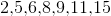Explanation:

To find mean, simply total the numbers and divide by 7.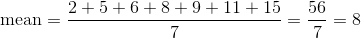### Example Question #2785 : Act Math

Find the mean of the following set of numbers: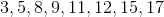Explanation:

To find the mean, simply sum all of the numbers and divide by how many there are.

Thus,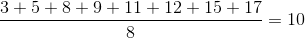### Example Question #2786 : Act Math

Find the mean of the following set of numbers: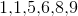Explanation:

To find the mean, simply sum up the numbers and divide by the amount there are. Thus,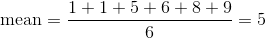### Example Question #61 : StatisticsFrom the menu above, what is the average price of a sandwich?  Round to the nearest cent.

$5.03$5.07

$5.11$5.09

$4.99 Correct answer:$5.07

Explanation:

Average  = Sum of the Options / # of Options

= (4.75 + 5.25 + 4.75 + 4.50 + 5.00 +5.25 + 6.00) / 7 = 35.50 / 7 = 5.07

### Example Question #1 : How To Find Median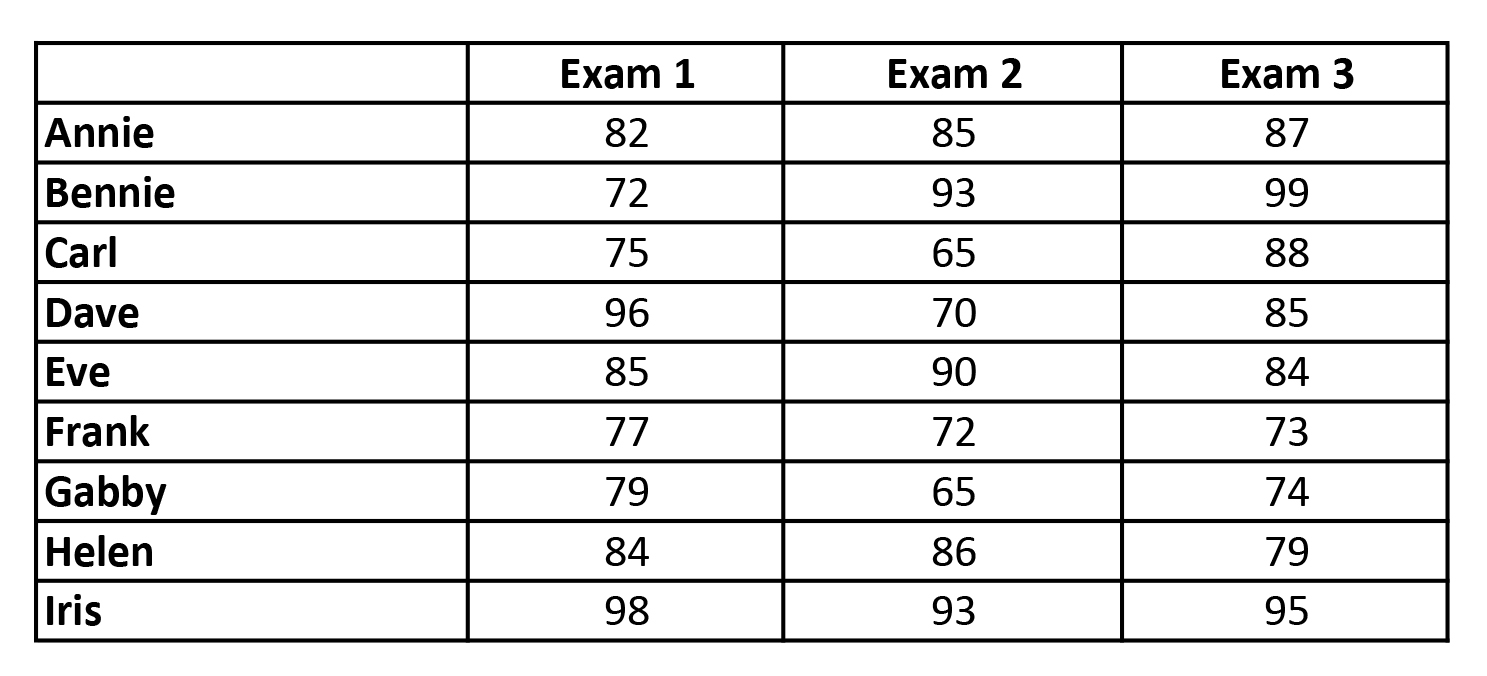Refer to the chart above. What is the Median of Exam 1?

84

85

82

79

83

82

Explanation:

To find the median, one must arrange all the heights from the lowest to the highest value and then pick the middle value.

All Values: 82 72 75 96 85 77 79 84 98

In Order: 72 75 77 79 82 84 85 96 98

Median: 82

### Example Question #2 : How To Find Median

Find the median of the given set of data:

{1,4,3,2,5,6,6}

1

3

4

2

6

4

Explanation:

First you must order the elements from least to greatest. In order, the set is {1,2,3,4,5,6,6}. There are 7 terms in this set, so the median will be the 4th term of the set, which happens to be 4.

### Example Question #3 : How To Find Median

Find the median of this set of data:

{1, 2, 3, 4, 5, 6}

4

3

4.5

2

3.5

3.5

Explanation:

When given an odd number of terms in a set, the middle term is the median.

However, if the number of terms is even, there are 2 middle terms, so the median is the average of the 2 middle terms. (3 + 4)/2 = 3.5

### Example Question #4 : How To Find Median

43, 83, 54, 35, 77,,If the median of the set of numbers is 54 and the mean is 56, which of the following could be the values ofand?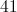and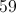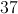and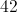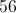and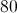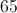and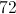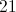and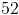andExplanation:

The median is the numerical value separating the higher half of the data sample from the lower half. Rearrange the data set by increasing value: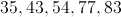In order for 54 to remain the median, either:

1. One of the variables must be greater than 54 and the other must be less than 54.

or

2. Both variables muat be equal to 54.

This eliminates all answer choices except 41 and 59. The mean of the data set including these two values is 56.

### Example Question #5 : How To Find Median

The following are the points scored on a test by students in a class:

5,9,11,11,12,13,13,13,18,20

Which of the following numbers is the mode?

12

14

13

15

11

13

Explanation:

13 is the correct answer because it occurs three times and occurs more than any other grade.

### Example Question #6 : How To Find Median

Find the median of the following set of numbers: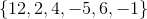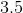Explanation:

Find the median of the following set of numbers:The median is the middle number in a group of numbers listed in order. To find the median, start by listing the numbers in increasing order: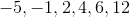Then find the middle number. In this case, there are two "middle numbers,"and, so the next step is to average them.

Therefore, the median is.

### All ACT Math Resources